# Test Prep Plan - Take a practice test

Take this practice test to check your existing knowledge of the course material. We'll review your answers and create a Test Prep Plan for you based on your results.
How Test Prep Plans work
1
2Based on your results, we'll create a customized Test Prep Plan just for you!
3Study smarter
Study more effectively: skip concepts you already know and focus on what you still need to learn.

# High School Geometry: Triangles, Theorems and Proofs Chapter Exam

Exam Instructions:

Choose your answers to the questions and click 'Next' to see the next set of questions. You can skip questions if you would like and come back to them later with the yellow "Go To First Skipped Question" button. When you have completed the practice exam, a green submit button will appear. Click it to see your results. Good luck!

### Page 1

#### Question 2 2. If the pictured triangles are congruent, what reason can be given?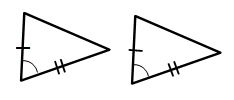#### Question 3 3. In the pictured triangles, what reason can we use to explain that angle QPR is congruent to angle SPT?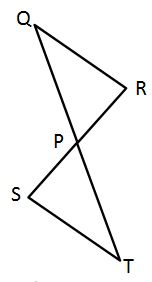#### Question 4 4. Triangle ABC is congruent to triangle XYZ. What reason can we use to explain why angle A is congruent to angle X?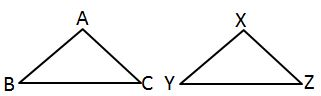#### Question 5 5. Which theorem or postulate can be used to establish congruence with the pictured triangles?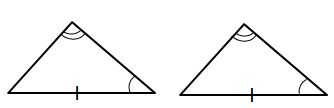### Page 2

#### Question 6 6. If the pictured triangles are congruent, what reason can be given?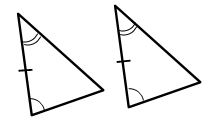#### Question 7 7. If the pictured triangles are congruent, what reason can be given?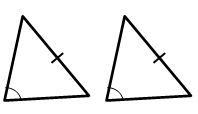#### Question 9 9. Which theorem can be used to establish congruence with the pictured triangles?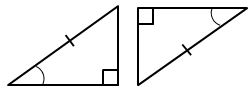#### Question 10 10. Study the given diagram. Find the value of x, the distance between T and E.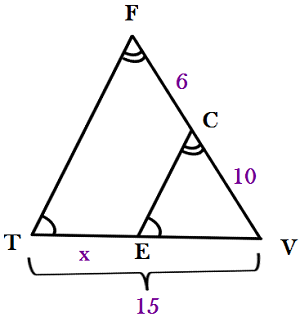### Page 3

#### Question 11 11. Given triangles DAR and KMR. What is the value of y?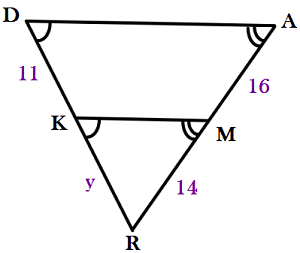#### Question 12 12. If triangle MNO is congruent to triangle STR, what must also be true?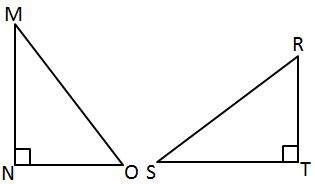#### Question 14 14. You are given that angle B and E are right angles and that the hypotenuses of the triangles are congruent. Which of these is additional information that will help you prove that triangle ABC is congruent to triangle DEF using the HA theorem?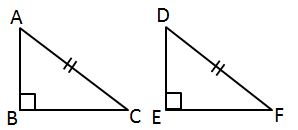#### Question 15 15. Triangle HYV and triangle AYB are similar by the AA similarity theorem. What is the value of x?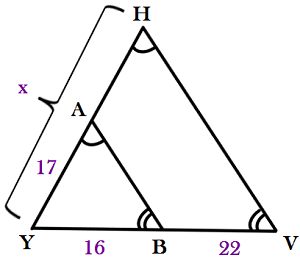### Page 4

#### Question 16 16. Find the measure of MN: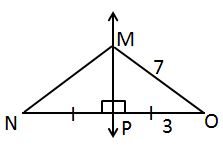#### Question 18 18. If the pictured triangles are congruent, what reason can be given?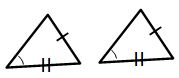#### Question 19 19. Which theorem or postulate can be used to establish congruence with the pictured triangles?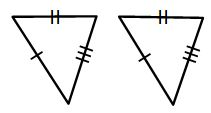#### Question 20 20. Which of the following represents the angle bisector theorem for the pictured triangle?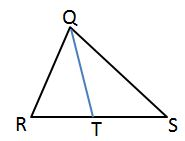### Page 5

#### Question 21 21. How can we explain that the pictured triangles are congruent?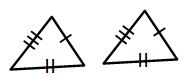#### Question 22 22. All of the following statements about the pictured triangle must be true, EXCEPT: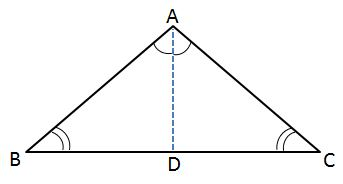#### Question 25 25. In the pictured triangle, MS is an angle bisector. What is the length of SO?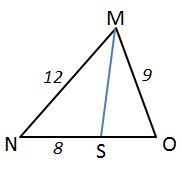### Page 6

#### Question 26 26. If the pictured triangles are congruent, what reason can be given?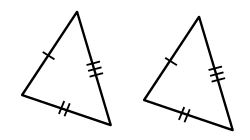#### Question 28 28. You want to try to prove that triangles PQS and PRS are congruent using the HA theorem. You are given that angle P and angle S are congruent and the triangles share a hypotenuse which means the hypotenuses are congruent. What additional information do you need in order to use the HA theorem?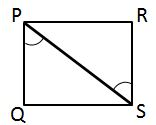#### Question 30 30. Which theorem can be used to establish congruence with the pictured triangles?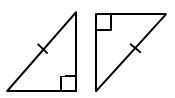#### High School Geometry: Triangles, Theorems and Proofs Chapter Exam Instructions

Choose your answers to the questions and click 'Next' to see the next set of questions. You can skip questions if you would like and come back to them later with the yellow "Go To First Skipped Question" button. When you have completed the practice exam, a green submit button will appear. Click it to see your results. Good luck!

Support